# Library release: visualize saliency map of deep neural network

Japanese is available at Qiita.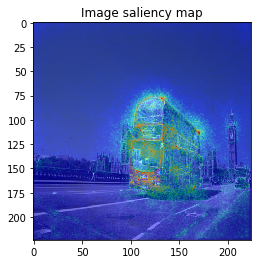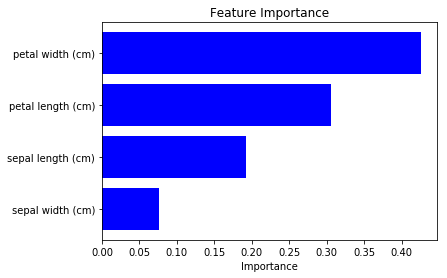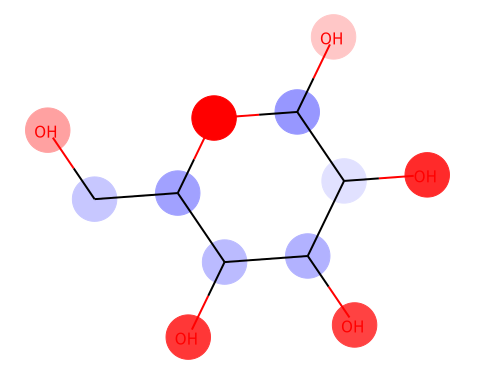From left: 1. Classification saliency map visualization of VGG16, CNN model. 2. iris dataset feature importance calculation of MLP model. 3. Water solubility contribution visualization of Graph convolutional network model.

## Abstract

Have you ever thought “Deep neural network is highly complicated black box, no one ever able to see what happens inside to result this output.”?

Even though NN consists of many layers and its mathematical analysis is difficult, there are some researches to show some saliency map like above images to understand model’s behavior or to get new knowledge for the dataset.

These saliency map calculation methods are implemented in Chainer Chemistry (even though the name contains “chemistry”, saliency module is available in many domains, as explained below). I will briefly explain how these work, and how to use it. You can also show these visualization figures after read this (a little bit long) article, enjoy!

It starts from theoretical explanation, followed by the code to use the module. Please jump to the “Examples” section if you just want to use it.

## What is reasoning of NN?

3 saliency calculation methods are implemented so far in chainer chemistry.

These methods calculate the contribution to the model’s prediction for each data.

※ Note that feature importance used in Random forest or XGBoost are calculated for the model. There is a difference that it is not calculated for “each data”.

This method calculates derivative of output y with respect to input x, as a input contribution to the output prediction.

$$s_i = \frac{dy}{dx_i}$$

Here, $$s_i$$ is the saliency score, which is calculated for each input data’s $$i$$-th element $$x_i$$. When the value of gradient is large for some element, the value change of this element results in big change of output prediction. So this element should have larger saliency (importance).

In terms of implementation, it is simply written as follows with chainer.

## Saliency module usage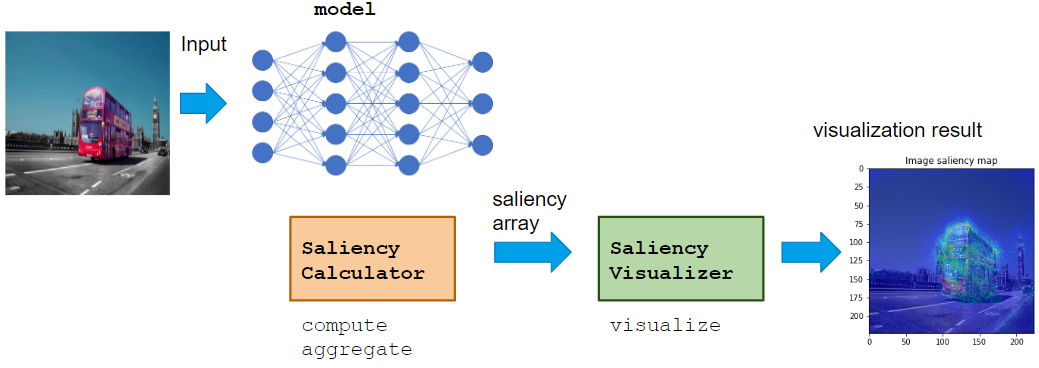Visualizer class visualizes calculated saliency score.

Calculator can be used with various NN model, which does not restrict the domain or application. Visualizer can be implemented to adopt Application for the proper visualization for the domain.

Basic usage flow is to call Calculator compute, aggregate -> Visualizer visualize

### Calculator class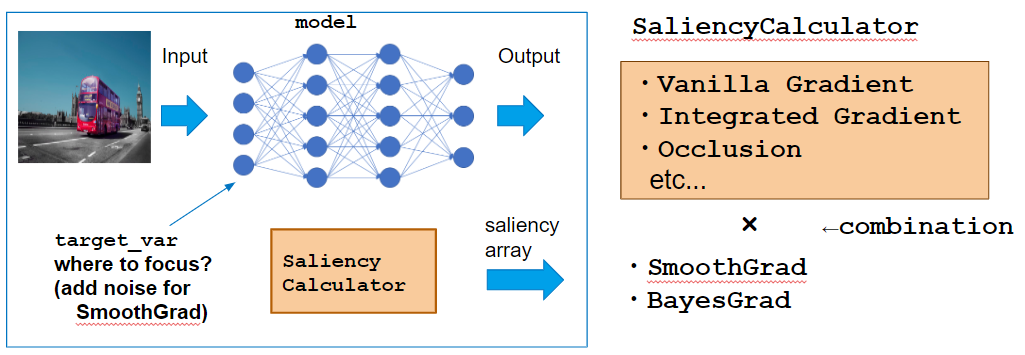Here I use GradientCalculator as an example which calcultes VanillaGrad explained above. Let’s see how to call each method.

#### Instantiation

Instance with passing model, which is the target neural network to calculate saliency.

#### compute method

compute method calculates “saliency samples” for each data x.

Here, M samples of saliency is calculated.

When calculating VanillaGrad, it suffices with M=1 since the calculation result of grad is always same. However, sampling is necessary when we consider SmoothGrad or BayesGrad.

I will explain SmoothGrad & BayesGrad to understand the notion of sampling.

Practically, VanillaGrad tends to show Noisy saliency map, so SmoothGrad suggests to change
input x to shift a small $$\epsilon$$, resulting input $$x + \epsilon$$ and calculate grad. We can take the average as the final saliency score.

$$s_{mi} = \frac{dy}{dx_i} |_{x=x+\epsilon_m}$$
$$s_{i} = \frac{1}{M} \sum_{m=1}^{M}{s_{mi}}$$

In the library, compute method calculates saliency sample $$s_{mi}$$, and aggregate method calculates saliency $$s_i = \frac{1}{M} \sum_{m}^{M} s_{mi}$$.

– project page: https://pair-code.github.io/saliency/

SmoothGrad changed input x by adding Gaussian noise, to take sampling. BayesGrad considers sampling along Neural Network parameter $$\theta$$, trained with dataset D, to get prediction posterior distribution $$y_\theta \sim p(\theta|D)$$ to take the sampling as follows:

$$s_{mi} = \frac{dy_\theta}{dx_i} |_{\theta \sim p(\theta|D)}$$

$$s_{i} = \frac{1}{M} \sum_{m=1}^{M}{s_{mi}}$$

– paper: https://arxiv.org/abs/1807.01985

#### aggregate method

This method “aggregates” M saliency samples $$s_{mi}$$ calculated by compute method, to obtain saliency $$s_i$$.

Aggregation methods differ by paper by paper, aggregate method in the library supports following 3 method.

‘raw’: simply take average
$$s_i = \frac{1}{M} \sum_{m}^{M} s_{mi}$$
‘abs’: take absolute average
$$s_i = \frac{1}{M} \sum_{m}^{M} |s_{mi}|$$
‘square’: take squared average
$$s_i = \frac{1}{M} \sum_{m}^{M} s_{mi}^2$$

### Visualizer class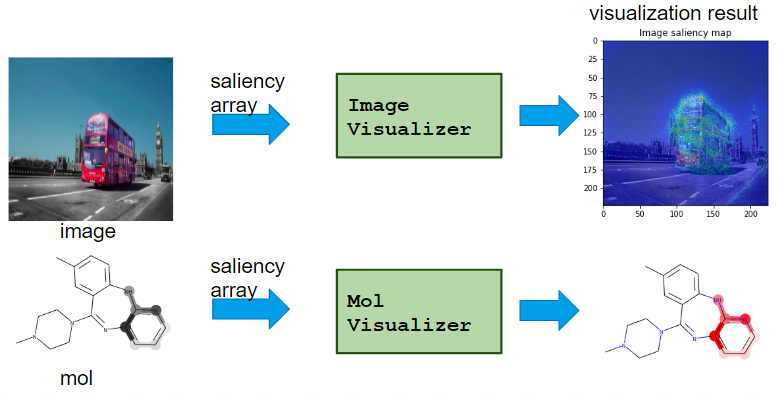It visualizes saliency from Calcualtor class.

TableVisualizer: plot feature importance for each table data
ImageVisualizer: plot saliency map of image
MolVisualizer: plot saliency map of molecule

As shown, Visualizer differs for each application.

#### visualize method

Visualizer plots figure with visualize method.

Note that Calculator class calcultes saliency with batch, but visualizer visualizes one data, so you need to specify it.

The figure can be saved by setting save_filepath argument.

## Examples

It was a long explanation,,, now let’s use it!

### Table data application: calculate feature importance

Neural Network is MLP (Multi Layer Parceptron), Dataset is iris dataset provided by sklearn.

iris dataset is to classify 3 flower species ‘setosa’, ‘versicolor’, ‘virginica’, from 4 features ‘sepal length (cm)’, ‘sepal width (cm)’, ‘petal length (cm)’, ‘petal width (cm)’.

Model’s training code is omitted (please refer the code on github). After training the model, we can use saliency module.

First, use Calculator compute -> aggregate to calculate saliency.

Second, use Visualizer visualize method to plot figure.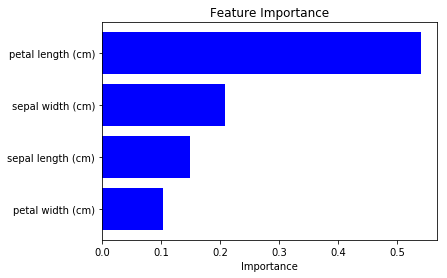We can see how the each feature contributes to the final output prediction loss.

We saw saliency for 0-th data above, now we can calculate average along dataset to show feature importance for all data (which roughly corresponds to model’s feature importance).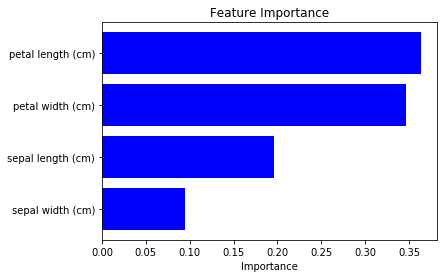We can see “petal length” and “petal width” are more important. (note that the result differs according to the model’s training condition, be careful.)

To check above result is plausible, I tried to plot feature impotance of Random Forest from sklearn (code).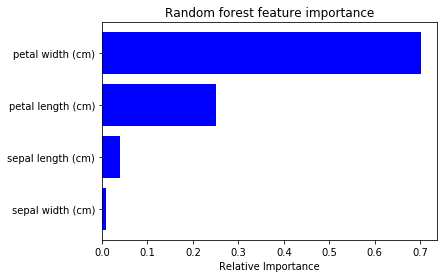Even though the absolute importance value differs, its order is same. So I feel the saliency calculation of NN is also useful for feature selection etc 🙂

### Image data: show saliency map for classification task

Training CNN takes time, so I will use pre-trained model. I will use VGG16 model provided by Chainer this time.

classes is 1000 class correct label as follows:Let’s try prediction at first.

When we see the result, 1-st image is correctly predicted as Basketball, 2nd image is predicted as trailer truck though it is actually bus, 3rd image is predicted as basenji (ImageNet contains various dog’s species as label, I do not know this is indeed correct or not…).

So let’s proceed to saliency calculation. This time, I will calculate saliency for “why predicting the label of top prediction”, not for the ground truth label. For example in 2nd image, we calculate saliency for why the CNN model predicted “trailer truck”, so the ground truth label (and the model predicts correct label or not) is not related.

I can set output_var as “softmax cross entropy between top prediction label” (instead of ground truth label).

Once eval_fun is defined, we can follow usual step: Calculator compute -> aggregate, ImageVisualizer visualize, to see the result.

We set ch_axis=2 in aggregate method, this is different from usual (minibatch, ch, h, w) image shape, because sampling_axis is added in front

ImageVisualizer visualization result is as follows: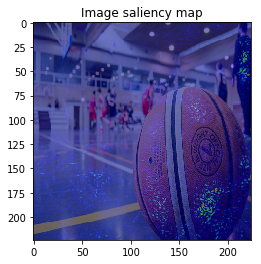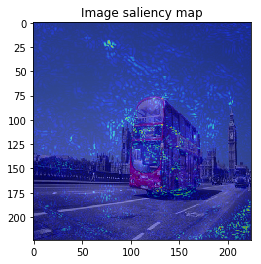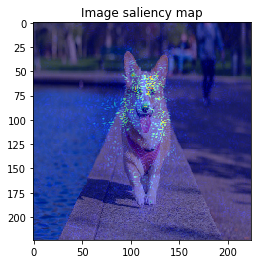It looks the model focuses on right place,,, but it is too noisy to see the result.

Next, let’s calculate SmoothGrad. We can set noise_sampler argument in Calculator compute method.

aggregate, visualize methods are same with VanillaGrad.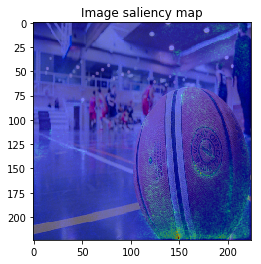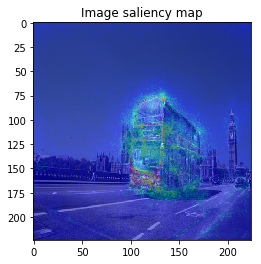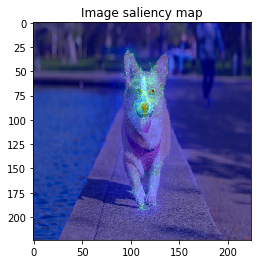The figure looks much better, we can see model focuses on the edge of objects.

At last, we will try BayesGrad. It requires that the model has stochastic operation. This time, VGG16 has dropout operation so it is applicable.

To calculate BayesGrad, we only need to set train=True in Calculator compute method. Chainer automatically enables dropout so that output is different in each samples, results that we can calculate saliency samples (gradient) for prediction distribution.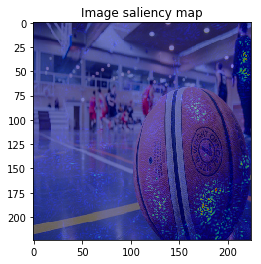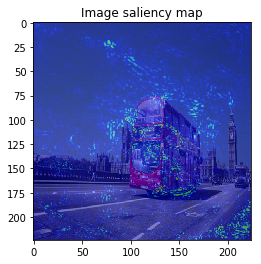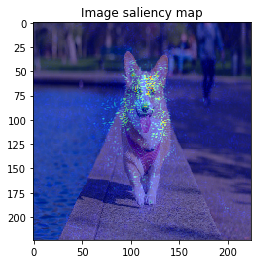This time, the result is similar to VanillaGrad.

When I try combining both SmoothGrad & BayesGrad, the result are as follows: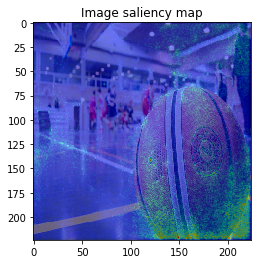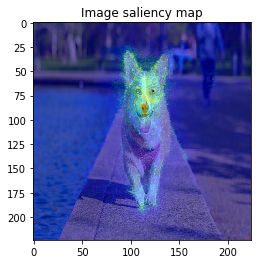### Molecule data: plot property contribution map for regression task

For regression task, we can calculate saliency to consider its sign, to show that the input contributes to positive or negative to the prediction.

In this last example, I will use Graph convolution model in Chainer Chemistry, to visualize water solubility contribution for each atom.

ESOL dataset is used for water solubility dataset.

After training the model (see repository for the code), we can proceed to visualization.

This time, we want to focus on contribution to the output prediction instead of loss. So we can define eval_fun to set output_var as predictor‘s output.

Also, we need to take care that input x is label of the node, gradient is not propagated until this input, we need to adopt gradient of the variable after embed layer, which is hidden layer’s variable.

In this kind of case, to set target_var as intermediate variable in the model, we can use VariableMonitorLinkHook.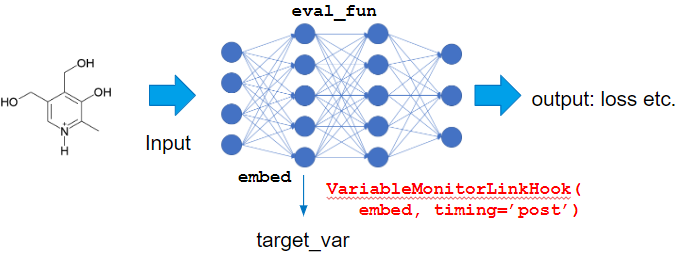I use IntegratedGradientsCalculator this time, to calculate saliency:

Visualization results are as follows,Red color shows the positive effect on solubility (Hydrophilic), blue color shows the negative effect on solubility (Hydrophobic).

Above figure matches the common sense of Hydrophilic effects usually occurs at polarization exists (OH), and we can see Hydrophobic effects where C-chain continues.

## Conclusion

I introduced saliency module, which is highly flexible and applicable to any domain.

You can try all the examples with few machine resources, only with CPU, so please try!! (Saliency map visualization of image uses pre-trained model so only inference is necessary).